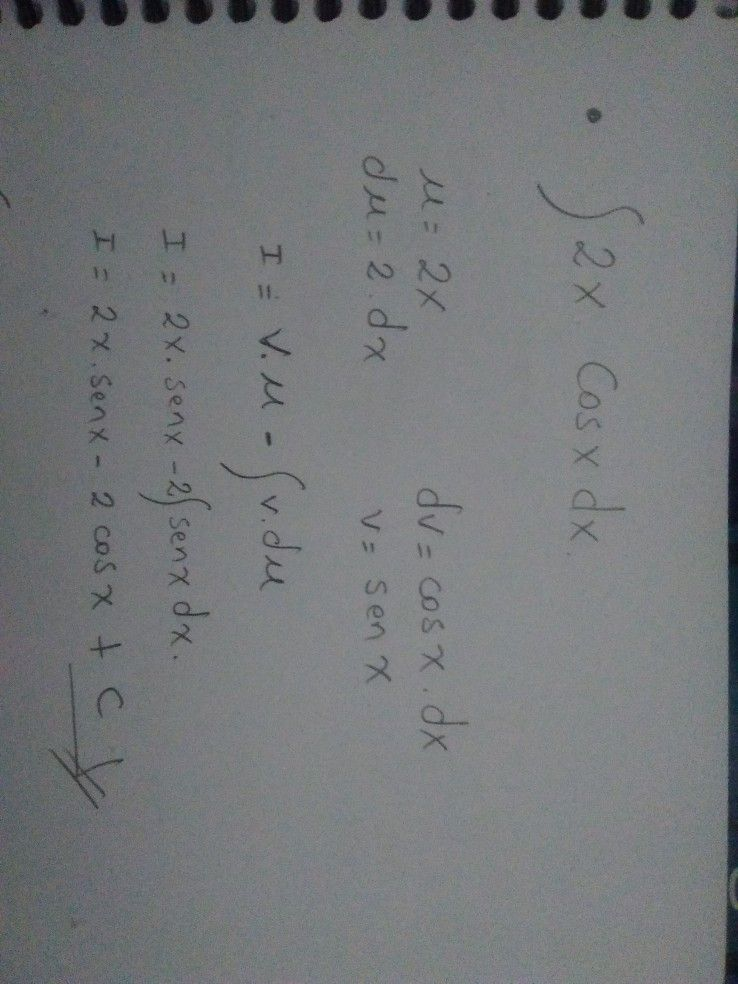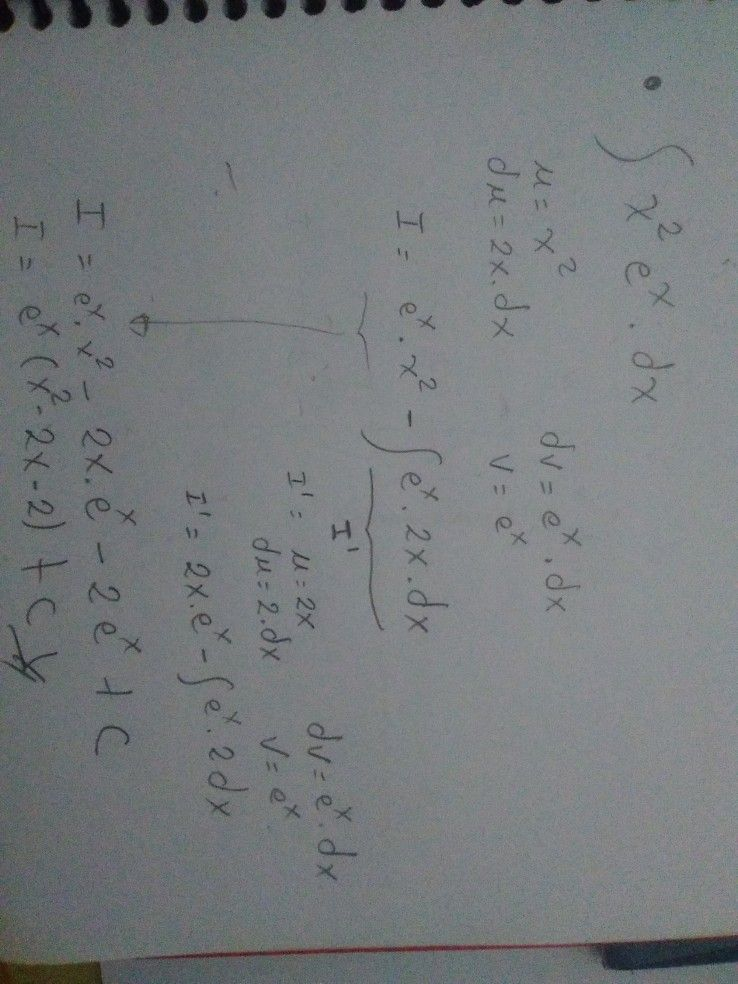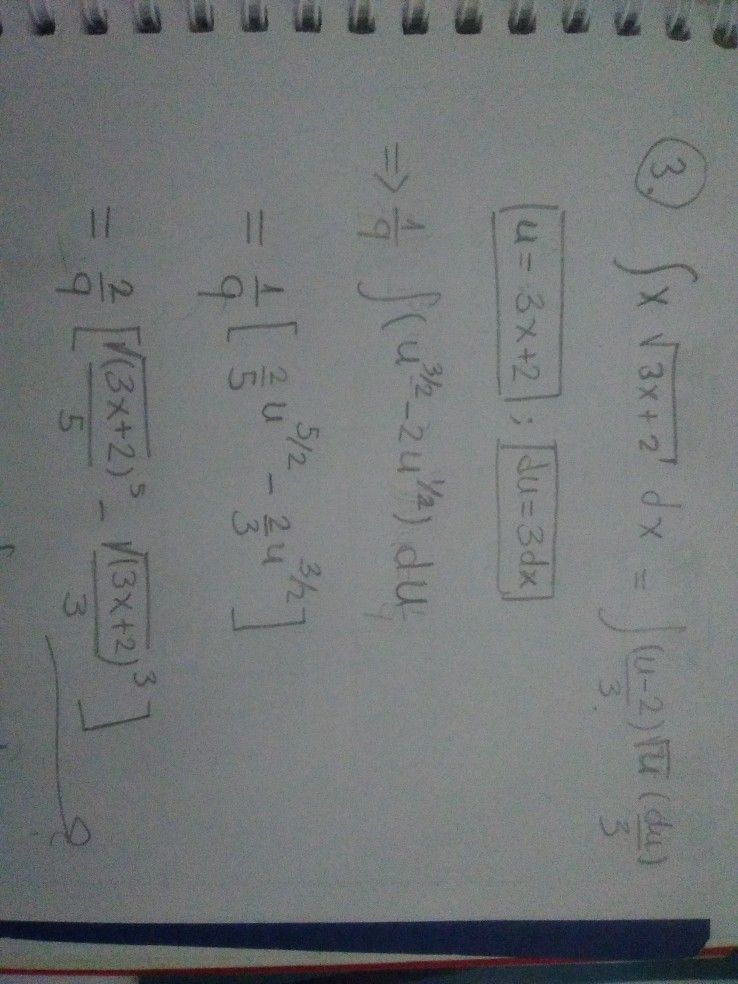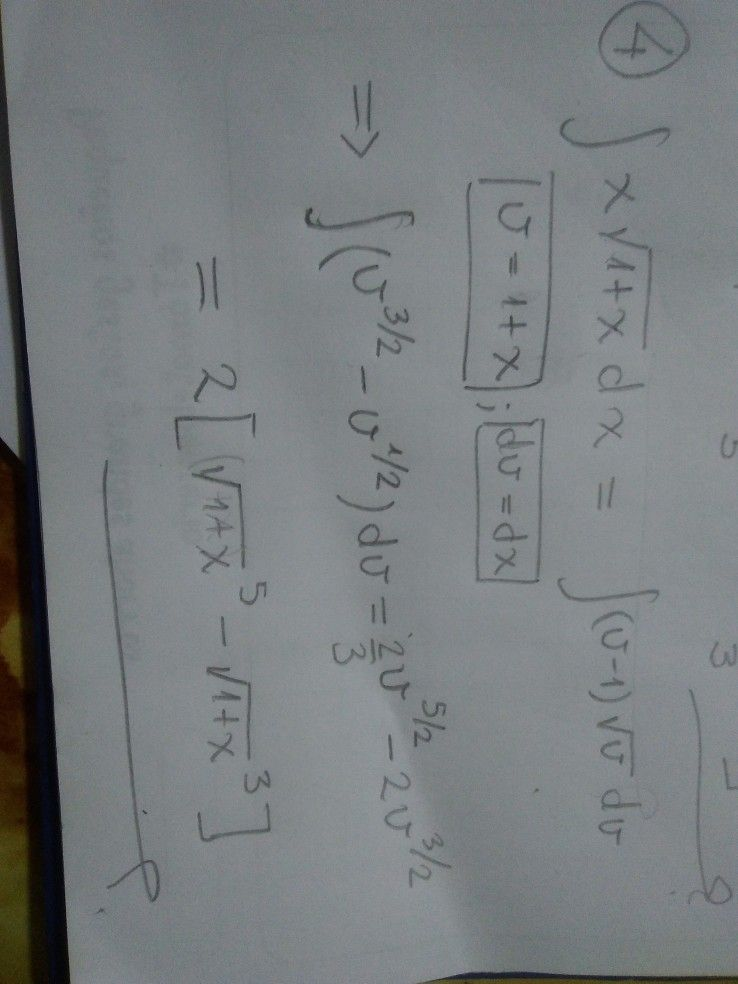Símbolos
Problema$y\int 2\times \left(00\times$ $d\times$ $3\int \times \sqrt{3\times +2}$ $c^{1}x$ $1\int$ $x^{2}$ $e^{\times }$ $dx$ $-$ $4\int$ $x$ $\sqrt{1+} \times dx$
Bachillerato
Cálculo
Búsquedas: 105
SoluciónProfesor de Qanda - LuisArturoEstudiante
muchas gracias este solo falto la cuarta es la que menos entiendoProfesor de Qanda - LuisArturoEstudiante
Gracias me ayudaste mucho